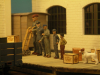# Figure Logbook January 28th 2011

## FiguresA few examples of figures in different scales. From Left: 1= 7/8in at 1:13.7, 2=1:16, 3=1:18, 4=1:20.3, 5=1:24log 110128 -- From Left; Figure 1=1/13.7, 2=1/16, 3=1/18, 4=1/20.3, 5=1/24log 110128 -- From Left; Figure 1=1/13.7, 2=1/16, 3=1/18, 4=1/20.3, 5=1/24log 110128 -- From Left; Figure 1=1/13.7, 2=1/16, 3=1/18, 4=1/20.3, 5=1/24

## another example

7/8in skip next to a 16mm scale skip from Binnie Engineering.log 110206 -- 7/8in skip next to a 16mm scale skip from Binnie Engineering